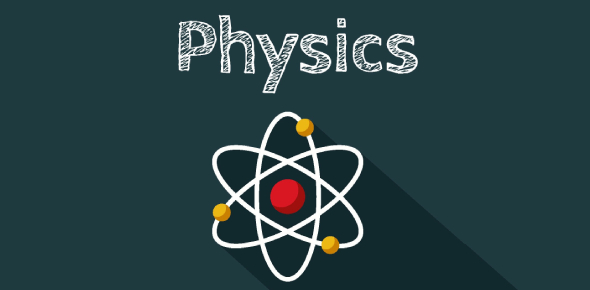# Take The Physics Trivia Quiz Questions To Pass!

25 Questions | Attempts: 449
ShareSettings.

• 1.
State and prove Gauss's Law in electrostatics and express the law in differential forms.(Give brief answers)
• 2.
Which techniques you will use to motivate the students?
• 3.
What would you do if the whole class is not getting the concept?
• 4.
How can you make learning fun for the students?
• 5.
Why should we consider you for the teaching job in our school?
• 6.
Waves travels on water surface through _____________________
• A.

Molecules

• B.

Atoms

• C.

Disturbance

• D.

Mass

• 7.
If the object is placed between f and 2f of convex lens, the image will be formed at ____________________.
• A.

At 2f

• B.

Beyond 2f

• C.

At f

• D.

Between f and 2f

• 8.
The distance between two point charges is 20cm. if the distance is reduced to 10cm, the Coulomb force would become____________________.
• A.

Half

• B.

Two times

• C.

One fourth

• D.

Four times

• 9.
In hot summer days reflection of water is seen on the road due to ______________.
• A.

Reflection

• B.

Refraction

• C.

Total internal reflection

• D.

Dispersion

• 10.
What is the induced in the coil when there is a relative motion between the coils of magnet?
• A.

Lines of force

• B.

Direct current

• C.

Emf

• D.

Alternating current

• 11.
The half-life of radium is 1600 years. How much of 120g of radium will be left after 4800 year?
• A.

120g

• B.

60g

• C.

30g

• D.

15g

• 12.
The relation between  linear  expansion  and  volume  expansion  is  ____________.
• A.

β = α/2

• B.

α = 3 β

• C.

β = 3 α

• D.

α = β/2

• 13.
A galvanometer is converted into ammeter when we connect a ____________
• A.

High resistance in series

• B.

High resistance in parallel

• C.

Low resistance in series

• D.

Low resistance in parallel

• 14.
The interval of 200µs is equivalent to_____________________.
• A.

0.2 sec

• B.

0.02sec

• C.
• D.
• 15.
If the capacitance of a capacitor is 10μF and potential difference is 2 volts then charge stored is _______________.
• A.

20 coulomb

• B.

200 coulomb

• C.
• D.
• 16.
Fahrenheit equivalent of 0K is__________________________.
• A.

– 273F°

• B.

– 459F°

• C.

0F°

• D.

100F°

• 17.
The work done will be zero when the angle between the force and the distance is ______________________.
• A.

45°

• B.

60°

• C.

90°

• D.

180°

• 18.
If mass of both the bodies is 1Kg and distance between their centers is 1m then gravitational force will be:
• A.

G

• B.

G

• C.

V

• D.

None

• 19.
For the propagation of signals in our body _________________energy is used.
• A.

Heat

• B.

Electrical

• C.

Chemical

• D.

Mechanical

• 20.
If the zero of the circular scale is below the horizontal line the zero error is to be_______________
• A.

• B.

Subtracted

• C.

Multiplied

• D.

Divided

• 21.
When trivalent impurity is added then ________________ is produced.
• A.

Free electron

• B.

Bound electron

• C.

Hole

• D.

Electron

• 22.
When current is passing through a solenoid it becomes______________________
• A.

Magnet

• B.

Permanent magnet

• C.

Temporary Magnet

• D.

None of these

• 23.
For scientific notation internationally accepted practice is that there should be ______________digit before the decimal point.
• A.

One

• B.

Two

• C.

Three

• D.

No

• 24.
A small steel ball is dropped from a low balcony. Ignoring air resistance, which statement describes its motion?
• A.

It falls with constant acceleration.

• B.

It falls with constant speed.

• C.

It falls with decreasing acceleration.

• D.

It falls with decreasing speed

• 25.
Which of the following is a unit of density?
• A.
• B.
• C.
• D.

## Related TopicsBack to top
×

Wait!
Here's an interesting quiz for you.